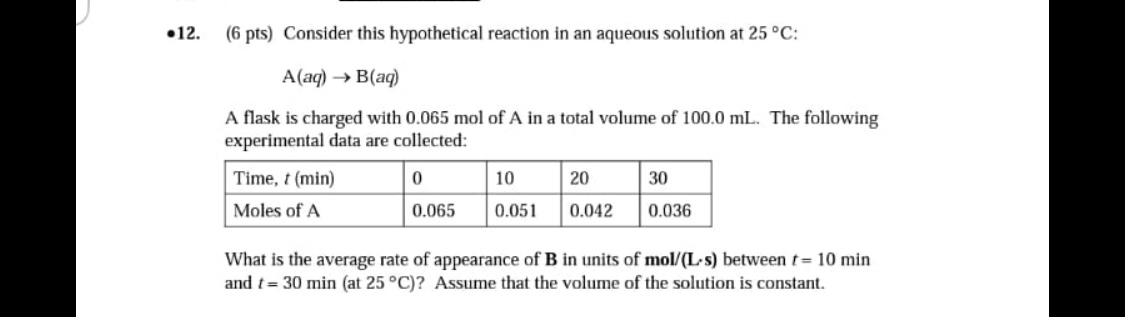# Consider this hypothetical reaction in an aqueous solution at 25 C: A(aq) -> B(aq). A flask charged with 0.065 mol of A in a total volume of 100.0 mL. The following experimental data are collected: What is the average rate of appearance of B in units of mol/(Ls) between t = 10 min and t = 30 min (at 25 C)? Assume that the volume of the solution is constant.